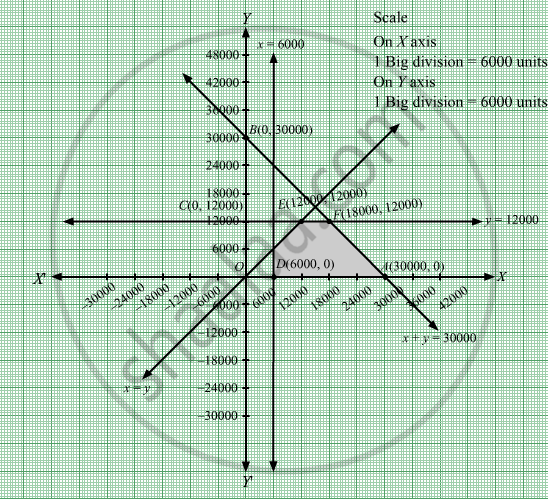# Maximize Z = 7x + 10y Subject to X + Y ≤ 30000 Y ≤ 12000 X ≥ 6000 X ≥ Y X , Y ≥ 0 - Mathematics

Maximize Z = 7x + 10y
Subject to

$x + y \leq 30000$
$y \leq 12000$
$x \geq 6000$
$x \geq y$
$x, y \geq 0$

#### Solution

We have to maximize Z = 7x + 10y
First, we will convert the given inequations into equations, we obtain the following equations:
x y = 30000,y = 12000, = 6000, x = yx = 0 and y = 0.

Region represented by x y ≤ 30000:
The line x y = 30000 meets the coordinate axes at $A\left( 30000, 0 \right)$ and $B\left( 0, 30000 \right)$  respectively. By joining these points we obtain the line x y = 30000.
Clearly (0,0) satisfies the inequation x y ≤ 30000. So,the region containing the origin represents the solution set of the inequation x y ≤ 30000.

The line y = 12000 is the line that passes through C(0,12000) and parallel to x axis.

The line x = 6000 is the line that passes through (6000, 0) and parallel to y axis.

Region represented by x ≥ y
The line x = y is the line that passes through origin.The points to the right of the line x= y satisfy the inequation x ≥ y.
Like by taking the point (−12000, 6000).Here, 6000 > −12000 which implies y > x. Hence, the points to the left of the line x = y will not satisfy the given inequation x ≥ y.

Region represented by x ≥ 0 and y ≥ 0:
Since, every point in the first quadrant satisfies these inequations. So, the first quadrant is the region represented by the inequations x ≥ 0 and ≥ 0.

The feasible region determined by the system of constraints, x y ≤ 30000, y ≤ 12000, x ≥ 6000, x   y , x ≥ 0 and y ≥ 0 are as follows:The corner points of the feasible region are D(6000, 0), $A\left( 3000, 0 \right)$ , $F\left( 18000, 12000 \right)$ and  $E\left( 12000, 12000 \right)$ .

The values of Z at these corner points are as follows:

 Corner point Z = 7x + 10y D(6000, 0) 7 × 6000 + 10 × 0 = 42000 $A\left( 3000, 0 \right)$ 7× 3000 + 10 × 0 = 21000 $F\left( 18000, 12000 \right)$ 7 × 18000 + 10 × 12000 = 246000 $E\left( 12000, 12000 \right)$ 7 × 12000 + 10 ×12000 = 204000

We see that the maximum value of the objective function Z is 246000 which is at  $F\left( 18000, 12000 \right)$  that means at = 18000 and y = 12000.
Thus, the optimal value of Z is 246000.

Concept: Graphical Method of Solving Linear Programming Problems
Is there an error in this question or solution?

#### APPEARS IN

RD Sharma Class 12 Maths
Chapter 30 Linear programming
Exercise 30.2 | Q 9 | Page 32